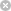RTXI  2.1msmrcost.cpp
Go to the documentation of this file.
1 //
2 // file = msmrcost.cpp
3
4 // computes cost (in terms of arithmetic operations)
5 // of multistage decimator and interpolator implementations
6 //
7 #include <fstream>
8 #include <iostream>
9 #include <math.h>
10
11 #include "filtmath.h"
12 #include "msmrcost.h"
13
14 void
15 MultistageMultirateCost(int num_stages, int* stage_factor,
16  double highest_samp_rate, double passband_edge_freq,
17  double passband_ripple, double stopband_ripple,
18  int num_taps_must_be_odd)
19 {
20  double delta_p, d_sub_inf;
21  int overall_rate_factor, stage;
22  double high_stage_samp_rate, low_stage_samp_rate;
23  double trans_width, stopband_edge_freq;
24  double total_mult_rate;
25
26  int* num_taps = new int[num_stages];
27  double* mult_rate = new double[num_stages];
28
29  delta_p = passband_ripple / num_stages;
30  d_sub_inf = DSubInf(delta_p, stopband_ripple);
31  std::cout << "D_inf is " << d_sub_inf << std::endl;
32
33  overall_rate_factor = 1;
34  for (stage = 0; stage < num_stages; stage++)
35  overall_rate_factor *= stage_factor[stage];
36
37  stopband_edge_freq = highest_samp_rate / overall_rate_factor / 2.0;
38
39  high_stage_samp_rate = highest_samp_rate;
40  total_mult_rate = 0.0;
41  for (stage = 0; stage < num_stages; stage++) {
42  low_stage_samp_rate = high_stage_samp_rate / stage_factor[stage];
43  trans_width = low_stage_samp_rate - stopband_edge_freq - passband_edge_freq;
44  std::cout << "trans_width = " << trans_width << std::endl;
45  num_taps[stage] = (int)ceil(d_sub_inf * high_stage_samp_rate / trans_width);
46  if ((num_taps[stage] % 2 != 1) && num_taps_must_be_odd)
47  num_taps[stage]++;
48
49  mult_rate[stage] = num_taps[stage] * low_stage_samp_rate / 2.0;
50  total_mult_rate += mult_rate[stage];
51
52  std::cout << "num taps for stage " << stage << " = " << num_taps[stage]
53  << std::endl;
54  std::cout << "mult rate = " << mult_rate[stage] << std::endl;
55  high_stage_samp_rate = low_stage_samp_rate;
56  }
57  std::cout << "total mult rate = " << total_mult_rate << std::endl;
58  delete[] num_taps;
59  delete[] mult_rate;
60  return;
61 }
double DSubInf(double delta_p, double delta_s)
Definition: filtmath.cpp:15
void MultistageMultirateCost(int num_stages, int *stage_factor, double highest_samp_rate, double passband_edge_freq, double passband_ripple, double stopband_ripple, int num_taps_must_be_odd)
Definition: msmrcost.cpp:15